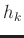## Data alignment using local similarity

Matching datasets involves aligning similar waveforms between two datasets. Whether aligning two logs from different wells or aligning a modeled synthetic seismogram with a seismic trace, we focus on matching a response that corresponds to similar lithologies between the two datasets or a common relative geologic time. In comparing two datasets, our purpose is to estimate the warping function,, required to align one dataset,, to a reference dataset,:(1)

We can represent the warping functionas follows,(2)

Thedenotes the original independent axis andis the shift required to match the datasets as defined in Equation 1.

We estimate the warping shiftsby using LSIM method based on the correlation coefficient, which can be used to quantify the quality of the match between datasets (Hampson-Russell, 1999). The LSIM method begins with the observation that the correlation coefficient only provides one number to describe the match; however, we are interested in understanding the local changes in the datasets' similarity. Therefore, the LSIM method computes local similarity, which is a function of time,. The square ofcan be split into a product of two factors (Fomel, 2007a):(3)

whereandare regularized least-squared inverses (Appendix A). This problem is posed as a regularized inversion where regularization operator is defined using shaping regularization and designed to enforce smoothness (Fomel, 2007b). To visualize LSIM, the inversion is calculated for a series to shifts. The results of this calculation are accumulated and displayed on a `similarity scan' as shown in Figures 5 and 7 in the following synthetic example. From the similarity scan, we automatically pick the series of shifts along the entire length of the reference dataset that optimally aligns the two datasets (Fomel and Jin, 2009).

To illustrate the alignment of two datasets using local similarity, we use two examples based on the simple model shown in Figure 3. In our first example, we apply a 40ms shift to the modeled synthetic seismogram and use LSIM to estimate the shifts to realign the shifted synthetic model with the original synthetic model (Figure 4). Estimation of the shifts for the first example is visualized in a local similarity scan shown in Figure 5. In our second example, we add 15% random noise to the reflectivity model and convolve the noisy reflectivity with a 30Hz Ricker wavelet to create a noisy reference trace. LSIM is used to estimate the shifts to realign the shifted synthetic model with the noisy reference trace in Figure 6. Estimation of the shifts for the second example is visualized in a local similarity scan shown in Figure 7. From our synthetic examples, we observe that shifts can be accurately estimated to align a modeled seismogram with both a noise-free and noisy reference seismograms. We use shifts estimated from local similarity to align multiple well logs and perform seismic well ties.modelb
Figure 3.
Reflectivity series in time (left) convolved with a 30Hz Ricker wavelet to model a seismogram (right).shifted-matched
Figure 4.
Modeled seismogram (left), modeled seismogram shifted by 40ms (middle), re-aligned seismogram using the shifts estimated from the local similarity scan (right).scan
Figure 5.
Similarity scan and picked optimal shifts (white curve). Warm colors represent high similarity while cool colors represent low similarity.noise-matched
Figure 6.
Noisy reference trace (left), modeled seismogram shifted by 40ms (middle), re-aligned seismogram using the shifts estimated from the local similarity scan (right).scan-noise
Figure 7.
Similarity scan and picked optimal shifts (white curve). Warm colors represent high similarity while cool colors represent low similarity.2019-05-07